Courses

# Linear Algebra (Basic Level) - 1

## 10 Questions MCQ Test Question Bank for GATE Computer Science Engineering | Linear Algebra (Basic Level) - 1

Description
This mock test of Linear Algebra (Basic Level) - 1 for Computer Science Engineering (CSE) helps you for every Computer Science Engineering (CSE) entrance exam. This contains 10 Multiple Choice Questions for Computer Science Engineering (CSE) Linear Algebra (Basic Level) - 1 (mcq) to study with solutions a complete question bank. The solved questions answers in this Linear Algebra (Basic Level) - 1 quiz give you a good mix of easy questions and tough questions. Computer Science Engineering (CSE) students definitely take this Linear Algebra (Basic Level) - 1 exercise for a better result in the exam. You can find other Linear Algebra (Basic Level) - 1 extra questions, long questions & short questions for Computer Science Engineering (CSE) on EduRev as well by searching above.
QUESTION: 1

### A square matrix is singular whenever:

Solution:

If the rows (or columns) of a square matrix are linearly dependent, then the determinant of matrix becomes zero.
Therefore, whenever the rows are linearly dependent, the matrix is singular.

QUESTION: 2

### If A and B are real symmetric matrices of size n x n. Then, which one of the following is true?

Solution:

The matrix M is s aid to be sym m etric iff MT= M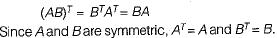QUESTION: 3

### Let a = (aij) be an n-rowed square matrix and I12 be the matrix obtained by interchanging the first and second rows of the n-rowed Identity matrix. Then AI12 is such that its first

Solution:

Let,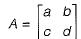I12 is the matrix obtained by inter-changing the first and second row of the identity Matrix I.
So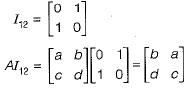AI12 is the matrix having first column same as the second column of A.

QUESTION: 4

The rank of the matrix given below is: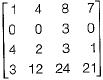Solution:

The given matrix is: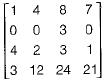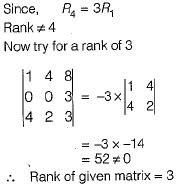QUESTION: 5

Consider the following determinant: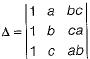Which of the following is a factor of Δ?

Solution:

The determinant of a matrix can’t be affected by elementary row operation
So,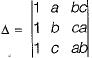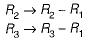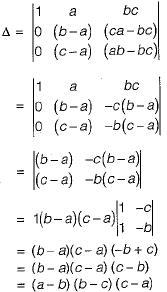So (a - b) is a factor of Δ.

QUESTION: 6

The rank of the matrix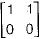is

Solution:

The given matrix isAbove matrix has only 1 independent row, so the given matrix has rank 1.

QUESTION: 7

The following system of equations: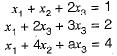Has a unique solution. The only possible value(s) for a is/are

Solution:

The augmented matrix for above system is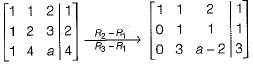Now as long as a - 5 ≠ 0,
rank (A) = rank (A | B) = 3
∴ a can take any real value except 5.

QUESTION: 8

If M is a square matrix with a zero determinant, which of the following assertion(S) is (are) correct?
S1: Each row of M can be represented as a linear combination of the other rows.
S2: Each column of M can be represented as a linear combination of the other columns.
S3: MX = O has a nontrivial solution.
S4: M has an inverse.

Solution:

S1 and S2:
Since M has zero determinant, its rank is not full i.e. if M is of size 3 x 3 , then its rank is not 3. So there is a linear combination of rows which evaluates to 0 i.e. k1R1 + k2R+...+knRn = 0 and there is a linear combination of columns which evaluates to 0 i.e.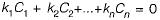Now any row Ri can be written as linear combination of other rows as: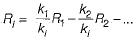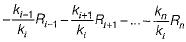Similar is the case for columns.
So S1 and S2 are true.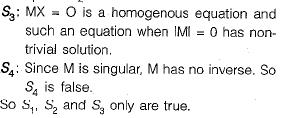QUESTION: 9

Consider the matrix as given below: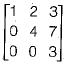Which one of the following options provides the CORRECT values of the eigenvalues of the matrix?

Solution:

Since the given matrix is upper triangular, its eigen values are the diagonal elements themselves, which are 1, 4 and 3.

QUESTION: 10

Consider the following 2 x 2 matrix A where two elements are unknown and are marked by ‘a’ and ‘b'. The eigenvalues of this matrix are -1 and 7. What are the values of ‘a’ and 'b'?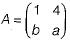Solution:

Trace = Sum of eigen values
1 + a = 6
⇒ a = 5
Determinant = Product of eigen values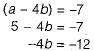⇒ b = 3
∴ a = 5, b = 3